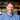# Swapping and Bitwise Operators

October 02, 2018

One of the simplest ways to swap two variables in programming is to create a temporary variable that holds a value while you iterate.

``````let swap = (i, j) => {
let temp = i;
i = j;
j = temp
}``````

Given two positions and an array, we are able to swap the values in constant time.

I wanted to know if there was an even simpler way. Was there a way that could be accomplished without the need of an extra variable.

It turns out that by using bitwise operators, there is.

# What is `^` and how does it work?

The `^` represents a bitwise operator called XOR. It is most commonly seen in engineering, mathematics, and computer science. The XOR operator returns a true (1) value whenever only one of the operands is true. In all other situations XOR returns false (0).Some notes about XOR in Javascript:

• Javascript will convert operands of a bitwise operator into binary (i.e. `x` and `y` in `x^y`).
• Javascript uses 32-bit integers in two’s complement format (more on this coming soon).

# Example Time!

If we use `bitwiseSwap` we can avoid defining a third variable!

``````var bitwiseSwap = (x,y) => {
if (x === y) {
return;
} else {
x = x ^ y;
y = x ^ y;
x = x ^ y;
}
}``````

Let’s now look at a simple example to bring this home and see how clever the `bitwiseSwap` approach actually is!

We’ll simplify the process by looking only at 4-bit numbers and swap 4 and 5.

1. Converting to binary: `0100 = 4` and `0101 = 5`
2. Check to ensure `x` does not equal `y` . Since they don’t, we can move on. (If they did, this process doesn’t work. Test it yourself to see why)
3. Setting `x = x^y`

``````0100 // x
0101 // y
----
0001``````

x now equals 1 (`0001`).

4. Setting `y = x^y`

``````0001 // x
0101 // y
----
0100``````

Holy magnolia, batman! `y` is now `0100`?!

But wait, it gets better. Let’s do our third and final mutation.

1. Setting x back to `x = x^y`

``````0001 // x
0100 // y
----
0101``````

# Conclusion

If space is limited, the bitwise operator can quickly (and accurately) two variables.

While logically sound, the temporary variable approach (listed above) is actually commonly preferred due to optimizations built into compilers. More importantly, the temporary variable approach is much more readable.

While this swap is not as practical, it does offer an interesting introduction to some of the potential uses of bitwise operators which are used to great effect within computer science and mathematics.Nota bene: both `swap` and `bitwiseSwap` are helper functions and will need to be modified in order to actually return, but their current forms are good for illustrative purposes.